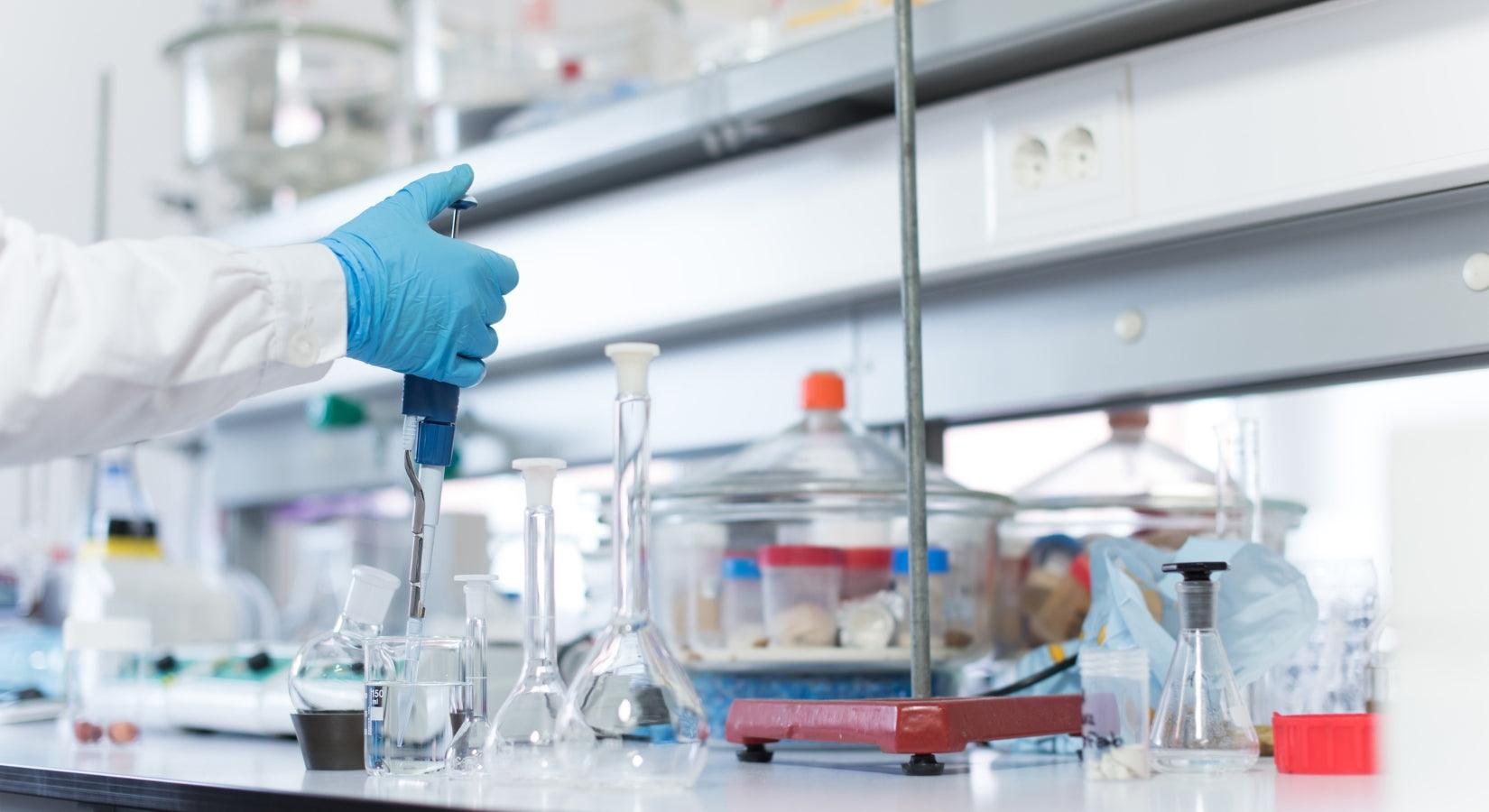HIRANUMA APPLICATION DATA Automatic Titrator Data No. J5 Apr. 5,2019
 Inorganic acids & Mixed acids Fractional determination of hydrochloric acid and sulfuric acid

## 1. Abstract

The mixed solution of hydrochloric acid and sulfuric acid works as strong acid, and also has the strong oxidizability and solvency. It is used as the surface treatment solution for metals, glass products, and semiconductors. Hydrochloric acid and sulfuric acid are strong acids, therefore the fractional determination by neutralization titration is difficult.
The total acids of hydrochloric acid and sulfuric acid in the mixed solution are determined first by neutralization titration in this report. Nitric acid is added to the sample solution continuously to adjust the pH. Finally, the concentration of hydrochloric acid is determined by precipitation titration, the concentration of sulfuric acid is calculated by subtracting the concentration of hydrochloric from the total acids concentration.
The example of fractional determination for hydrochloric acid and sulfuric acid with additional burets are introduced in this report.H₂SO₄ + 2NaOH → Na₂SO₄ + H₂O ・・・(1) HCl + NaOH → NaCl + H₂O ・・・(2) HCl + AgNO₃ → AgCl + HNO₃ ・・・(3)

## 2. Configuration of instruments and Reagents

 (1) Configuration of instruments Main unit : Hiranuma Automatic Titrator COM series Option : One buret, Peristaltic pump type dispenser Electrodes : Glass electrode GE-101B for total acids measurement Connect to IE-1.Silver-reference electrode AGR-811Z for hydrochloric acid measurement(Double junction type) Connect to IE-2 and RE-2. (2) Reagents Titrant : 0.1 mol/L Sodium hydroxide standard solution for total acids measurement0.1 mol/L Silver nitrate for hydrochloric acid measurement Additive solution : 2 mL of 1 mol/L Nitric acid solution for pH adjustment

## 3. Measurement procedure

 (1) Dispense 1 mL of sample into 30 mL DI water in a volumetric flask with volumetric pipette. Cool it to room temperature and dilute to 100 mL with DI water. (2) Dispense 10 mL of diluted sample solution into a 100 mL beaker with volumetric pipette. (3) Add 40 mL of DI water. (4) Immerse electrodes and start titration with 0.1 mol/L sodium hydroxide standard solution. (5) After the procedure (4), 2 mL of 1 mol/L nitric acid is automatically dispensed by optional dispenser. (6) Titrate with 0.1 mol/L silver nitrate standard solution with optional buret.

## 4. Measurement conditions and results

#### Examples of titration conditions

(1) Titration for total acids with sodium hydroxide standard solution (converted into hydrochloric acid)

 Cndt No 1 Method Auto Buret No. 1 Amp No. 1 D. Unit pH S-Timer 5 sec C.P. mL 0 mL T Timer 0 sec D.P. mL 0 mL End Sens 1000 Over mL 0 mL Max Vol. 20 mL Max Vol. Constant No. 1 Size 10 mL Blank 0 mL Molarity 0.1 mol/L Factor 1.004 K 1 L 0.01 Unit mol/L Formula (D-B)*K*F*M/(S*L) Decimal Places 3 Auto In Pram. None Mode No. 20 Pre Int 0 sec Del K 9 Del Sens 0 mV Int Time 2 sec Int Sens 3 mV Brt Speed 2 Pulse 40

K: Equivalent of NaOH to HCl
L: Dilution ratio

(2) Dispense 1 mol/L nitric acid

 Cndt No 2 Method Disp Buret No. 3 S-Timer 0 sec Disp Vol. 2 mL

(3) Titration for hydrochloric acid with silver nitrate standard solution

 Cndt No. 3 Method Auto Buret No. 2 Amp No. 2 D. Unit mV S-Timer 5 sec C.P. pH 0 mL T Timer 0 sec D.P. mL 0 mL End Sens 300 Over mL 0.2 mL Max Vol. 20 mL ConstantNo. 3 Size 10 mL Blank 0 mL Molarity 0.1 mol/L Factor 1.005 K 1 L 0.01 Unit mol/L Formula (D-B)*K*F*M/(S*L) Decimal Places 4 Auto In Pram. None Mode No. 6 Pre Int 0 sec Del K 2 Del Sens 0 mV Int time 3 sec Int Sens 3 mV Brt Speed 2 Pulse 40

(4) Calculation for sulfuric acid concentration

 Cndt No. 4 Method Calc ConstantNo. 4 Size 10 mL Blank 0 mL Molarity 0.1 mol/L Factor 1 K 1 L 0.01 Unit mol/L Formula (CA-CB)/2 Decimal Places 4 Auto In Pram. None

#### Measurement results

(1) Results of Total acids

Number of
measurement
Size
(mL)
Dilution
ratio
NaOH Titrant
Volume(mL)
Total acids (mol/L)
1 10 1/100 6.777 6.8041
2 10 1/100 6.776 6.8031
3 10 1/100 6.779 6.8061
Statistic
calculation
Avg. 6.804 mol/L
SD 0.002 mol/L
RSD 0.022 %

(2) Results of Hydrochloric acid and Sulfuric acid

Number of
measurement
Size
(mL)
Dilution
ratio
AgNO3 Titrant
volume (mL)
Hydrochloric acid
Conc. (mol/L)
Sulfuric acid
Conc. (mol/L)
1 10 1/100 0.683 0.6864 3.0589
2 10 1/100 0.680 0.6834 3.0599
3 10 1/100 0.682 0.6854 3.0603
Statistic
calculation
Avg. 0.685 mol/L 3.060 mol/L
SD 0.002 mol/L 0.001 mol/L
RSD 0.223 % 0.02 %

#### Examples of titration curvesMeasurement of total acids Measurement of hydrochloric acid

## 5. Note

(1) Fractional determination of hydrochloric acid and sulfuric acid
The concentration of hydrochloric acid is measured by the determination of chloride ion in this method.
This method cannot be applied to the sample containing chloride ion from other than hydrochloric acid.
(2) Control of titrant
The carbon dioxide gas absorber (soda lime) on reagent bottle has to be regularly exchanged because sodium hydroxide used for total acids determination readily absorbs carbon dioxide gas in the air.

Keywords： Fractional determination of hydrochloric acid and sulfuric acid, Neutralization titration,Precipitation titration

*Some measurement would not be possible depending on optional configuration of system.

Featured Instruments

View all